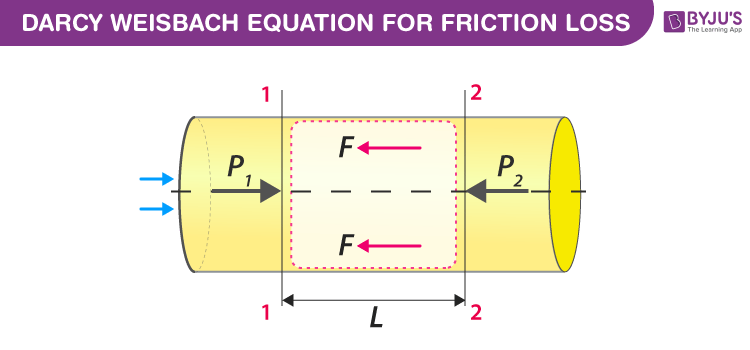Jet Set Go! All about Aeroplanes Jet Set Go! All about Aeroplanes

# Darcy Weisbach Equation Derivation - Explanation and Applications

## What Is Darcy Weisbach Equation?

It is an empirical equation in fluid mechanics named after Henry Darcy and Julius Weisbach. The Darcy Weisbach Equation relates the loss of pressure or head loss due to friction along the given length of pipe to the average velocity of the fluid flow for an incompressible fluid.

 $$\begin{array}{l}H_{F}=\frac{4fLv^{2}}{2gd}\end{array}$$

Where,

HF is the head loss or pressure loss.
f is the coefficient of friction or friction factor.
v is the velocity of incompressible fluid.
L is the length of the pipe.
d is the diameter.
g is the acceleration due to gravity. (g = 9.8m/s2)

## Derivation of Darcy Weisbach Equation### Step 1: Terms and Assumptions

Consider a uniform horizontal pipe with fixed diameter d and area A, which allows a steady flow of incompressible fluid.

For simplicity consider two sections; S1 and S2 of the pipe separated by the distance L.

At all the point of S1, The pressure is P1, velocity is V1.

At all the point of S2, the pressure is P2 and velocity is V2.

Consider the fluid flow as shown in figure(1) Thus, the pressure at S1is greater than the pressure at S2 i.e.,(P1>P2) This pressure difference makes the fluid flow along the pipe.

When fluid flows there will be a loss of energy due to friction. Thus we can apply Bernoulli’s principle.

#### Bernoulli’s principle

Bernoulli’s principle states that a decrease in the pressure or potential energy of the fluid increases the velocity/speed of the fluid flow or in other words, “For an incompressible fluid, the sum of its potential energy, pressure, and velocity remains constant.”

### Step 2: Applying Bernoulli’s principle

On applying Bernoulli’s equation in sections; S1 and S2 we get-

$$\begin{array}{l}P_{1}+\frac{1}{2}\rho v_{1}^{2}+\rho gh_{1}=P_{2}+\frac{1}{2}\rho v_{2}^{2}+\rho gh_{2}+H_{F}\end{array}$$
—–(1)

Where,

HF is the head loss due to friction

On dividing above equation (1) by 𝜌g we get-

$$\begin{array}{l}\frac{P_{1}}{\rho g}+\frac{v_{1}^{2}}{2g}+h_{1}=\frac{P_{2}}{\rho g}+\frac{v_{2}^{2}}{2g}+h_{2}+H_{F}\end{array}$$
——-(2)

For horizontal pipe (That is, the inlet of pipe and the outlet of the pipe are at the same level from the reference plane)

h1=h2.

Here, the diameter is uniform, for uniform diameter-

v1=v2

On substituting them, the equation(2) becomes-

$$\begin{array}{l}\frac{P_{1}}{\rho g}+\frac{v_{1}^{2}}{2g}+h_{1}=\frac{P_{2}}{\rho g} +\frac{v_{1}^{2}} {2g}+h_{1}+H_{F}\end{array}$$
$$\begin{array}{l}\Rightarrow \frac{P_{1}}{\rho g}=\frac{P_{2}}{\rho g}+H_{F}\end{array}$$
—–(3)

Thus, on rearranging equation (3) for the head loss we get-

$$\begin{array}{l}H_{F}= \frac{P_{1}}{\rho g}-\frac{P_{2}}{\rho g}\end{array}$$
$$\begin{array}{l}\Rightarrow H_{F}= \frac{P_{1}-P_{2}}{\rho g}\end{array}$$
$$\begin{array}{l}\Rightarrow H_{F}\rho g= P_{1}-P_{2}\end{array}$$
——-(4)

### Step 3: Find frictional resistance

Due to the combined effect of wet surface and surface roughness, the resistance is offered to the flow of fluid due to friction. As a result, speed is reduced. The Froude was the first person to observe the dependency of frictional resistance with surface roughness.

The frictional resistance is well expressed through Froude’s formula.

Let f’ be the frictional resistance per unit area(wet) per unit velocity.

Frictional resistance F = f’ × wet area × (velocity)2

= f’ × 2𝜋rL × v2

= f’ × 𝜋dL × v2

F = f’ × PL × v2 ——-(5)

[here, diameter; d=2r and perimeter; P=𝜋d]

### Step 4: Net force acting on the fluid at section S1 and S2

The net force is the sum of Force due to pressure at S1, S2, and fluid friction.

• At S1, pressure is given by-
• $$\begin{array}{l}P_{1}=\frac{F_{1}}{A}\end{array}$$

Which implies, The net force

$$\begin{array}{l}F_{1}=P_{1}A\end{array}$$

For our convenience consider the direction of the force due to pressure as +ve.

• At S2, the pressure is given by-
• $$\begin{array}{l}P_{2}=\frac{F_{2}}{A}\end{array}$$

Which implies, The net force

$$\begin{array}{l}F_{2}=P_{2}A\end{array}$$

here, the direction of the force due to pressure as -ve.

• Fluid Frictional force(F): It is a resistive force, thus the direction as -ve.
• Thus, resolving all the forces along the horizontal direction we get-

P1A – P2A – F = 0

P1A – P2A = F

(P1– P2)A = F

$$\begin{array}{l}\Rightarrow P_{1}-P_{2}=\frac{F}{A}\end{array}$$
——(6)

Substitute the values for F and (P1– P2) from equation (5) and (4) respectively.

$$\begin{array}{l}\Rightarrow \rho gH_{F}=\frac{f’PLv^{2}}{A}\end{array}$$

On rearranging the terms we get-

$$\begin{array}{l}H_{F}=\frac{f’PLv^{2}}{A \rho g}\end{array}$$
$$\begin{array}{l}H_{F}=\frac{f’}{\rho g}\times Lv^{2}\times \frac{P}{A}\end{array}$$
—–(7)

But,

$$\begin{array}{l}\frac{P}{A}=\frac{Wetted\;perimeter}{Area}=\frac{\pi d}{\frac{\pi }{4}d^{2}}=\frac{4}{d}\end{array}$$

Substituting

$$\begin{array}{l}\frac{P}{A}=\frac{4}{d}\end{array}$$
in equation(7),

$$\begin{array}{l}\left ( 7 \right )\Rightarrow H_{F}=\frac{f’}{\rho g}\times \frac{4Lv^{2}}{d}\end{array}$$
——-(8)

Now substitute

$$\begin{array}{l}\frac{f’}{\rho}=\frac{f}{2}\end{array}$$

Where,

f’ is a frictional resistance

⍴ is the density of the fluid.

f is the coefficient of friction

Because the fluid is incompressible, which means that with the application of the external force there will not be any change in the density.

$$\begin{array}{l}\left ( 8 \right )\Rightarrow H_{F}=\frac{f}{2g}\times \frac{4Lv^{2}}{d}\end{array}$$

Thus, On rearranging, we finally arrive at Darcy Weisbach Equation

 $$\begin{array}{l}H_{F}=\frac{4fLv^{2}}{2gd}\end{array}$$